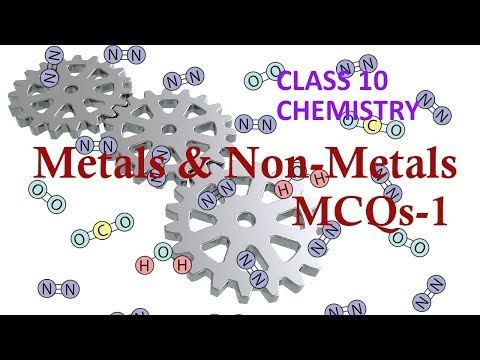## Pages

Showing posts with label class10-chemistry. Show all posts
Showing posts with label class10-chemistry. Show all posts

# CBSE Class 10 Chemistry - Carbon and Its Compounds - Very Short Answer Based Questions on Allotropes (Part -2)

Q1: What is the atomic number of a carbon atom?

Q2: How many valence electrons are present in a carbon atom?

Answer: 4 (electronic configuration is K:2 L:4)

Q3: What are allotropes?

Answer: The property due to which one element exists in more than one form, which differs in physical properties but has similar chemical properties. These forms of an element are called allotropes.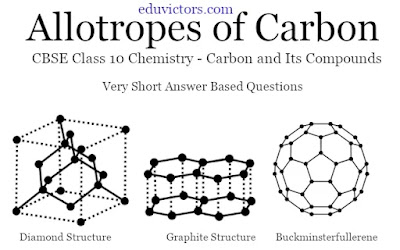# CBSE Class 8 Chemistry - Metals and Non-Metals (MCQs)

Q1: All metals are solids except:

(a) Sodium

(b) Calcium

(c) Mercury

(d) Copper

Q2: Which of the following metal does not react with dilute Hydrochloric acid?

(a) Iron

(b) Sodium

(c) Magnesium

(d) Copper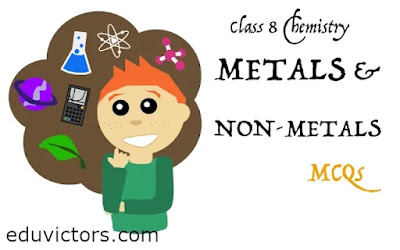# Class 10 Chemistry - Acid, Bases and Salts (Worksheet)

Fill in the blanks with suitable words

1. An acid is a compound which when dissolved in water gives _________ ions as the only _________ ions.

2. Higher the pH value of a solution, the more __________ it is.

3. pH value of lemon juice is _________ than 7.# Chemistry - Colours Of Compounds For CBSE Class 12, 11, 10 and 9

Salts/Compounds     Colour

1. Potassium dichromate, K₂Cr₂O₇ Orange

2. Potassium permanganate, KMnO₄ Violet

3. Ferric tetra-oxide, Fe₃O₄ Black

4. Ferrous sulphate, FeSO₄.7H₂O Green

5. Ferric sulphate, Fe₂(SO₄)₃ Yellow

6. Ferric hydroxide, Fe(OH)₂ Green# Class 10 - Chemistry - Periodic Classification Of Elements (Very Short Answer Based Questions)

Q1: What is the basis of classification of elements in the modern periodic table?

Q2: The Law of Octaves was applicable only upto which element?

Q3: Which important property did Mendleev use to classify the elements in the periodic Table?

Answer: He used atomic masses of elements in classifying elements.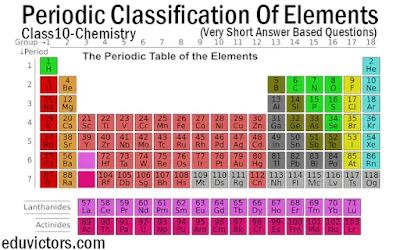## CBSE Class 10 - Chemistry - Acids, Bases and Salts - Assertion Reason Based Questions

Assertion and Reasoning based questions are a bit tricky. You should be able to distinguish whether the assertion is well connected to the reason or these are just random facts. Read both statements carefully, and check if both statements are factually or logically correct or not. If both are correct, then try to check if both are well connected to each other or not.

The questions below consist of statements of an Assertion and a Reason. Use the following key to choose an appropriate answer:

(a) Both A and R are true and R is the correct explanation of A.

(b) Both A and R are true but R is not the correct explanation of A.

(c) A is true but R is false.

(d) A is false but R is true.

Q1: Assertion (A) : Acids are those substances which turn blue litmus solution red.
Reason (R)    : Acids give more OH⁻ ions in aqueous solution.# CBSE Class 10 - Chemistry - Acids, Bases and Salts (Q & A) Part-1

Based on chemical properties, all compounds can be classified as acids, bases and salts. To identify acids and bases, indicators are used. This video talks about types of indicators i.e. natural, synthetic and olfactory. Examples of indicators and related questions are also covered.

Here follows the video: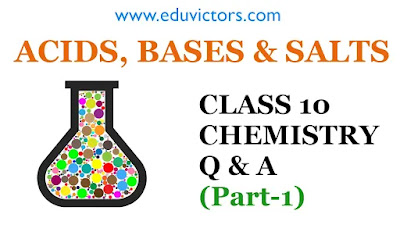# CBSE Class 10 - Chemistry - Chemical Reactions and Equations (Q & A) Part-4

The first chapter of Chemistry talks about chemical reactions and equations. In a chemical reaction, reactants are transformed into products. In this series, the part-4 video discusses:

- corrosion

- examples of corrosion (good vs bad)

- methods to prevent corrosion

- rancidity

- methods to prevent rancidity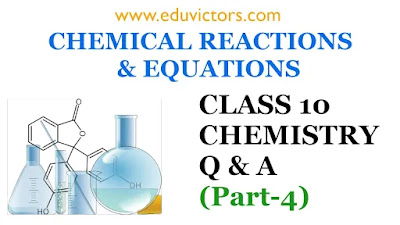# Class 10 Chemistry Sample Question Paper 2020-21

Learning is a continual process. To improve scores, the best way is to learn and practice. Solving question papers help students to evaluate their knowledge and familarise with different types of question patterns being asked in the examination.

Eduvictors provides CBSE Previous Year Papers and Sample Question Papers for Class 10 Maths, Science, Social, English, Hindi and Sanskrit with/without solutions to help students in their board exam preparation.

Here attached the Chemistry Sample Question Paper (2020-2021) for your self-evaluation.# Class 10 - SCIENCE - Question Bank - Very Short Answers Based Questions (Unsolved)

1. Why does not a wall immediately acquire a white colour when a coating of slaked lime is applied on it ?

2. Define displacement reaction with one example.

3. Identify the most reactive and least reactive metal : Al, K, Ca, Au.

4. Which of the following is a combination reaction and which is a displacement reaction ?

(a) Cl₂ + 2KI ——–> 2KCl + I₂

(b) 2K + Cl₂ ——–> 2KCl.

5. What is the difference between the following two reactions ?

(a) Mg + 2HCl ——–> MgCl2 + H2

(b) NaOH + HCl ——–> NaCl + H2O.

6. Identify the compound which is oxidised in the following reaction

H₂S + Br₂ ———–> 2HBr + S.

7. What happens chemically when quick lime is added to water ?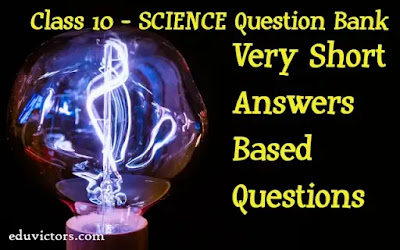# CBSE Class 10 - Chemistry - METALS AND NON-METALS (Very Short Answer Based Questions)

Q1. A green layer is gradually formed on a copper plate left exposed to air for a week in a  bathroom. What could this green substance be?

Answer. It is due to the formation of basic copper carbonate [CuCO₃.Cu(OH)₂].

Q2. Why are elements of Group IA i.e. H, Li, Na, K, Cs and Fr called alkali metals?

Answer. Because oxides of these elements dissolve in water to give alkali or soluble hydroxides.

Q3. A non-metal X exists in two different forms Y and Z. Y is the hardest natural substance,   whereas Z is a good conductor of electricity. Identify X, Y and Z.

Answer. ‘X’ is carbon, ‘Y’ is diamond as it is the hardest natural substance and ‘Z’ is graphite as   it is good conductor of electricity.# CBSE Class 10 Chemistry - Chapter 1: Chemical Reactions and Equations (Part-3)(Q & A)

The first chapter of Chemistry talks about chemical reactions and equations. In a chemical reaction, reactants are transformed into products. In this series, the part-3 video discusses types of chemical reactions.

Following questions are covered:

Q1: What are the important types of chemical reactions.

Q2: What are combination reactions? Give examples.

Q3: Hydrogen burns in oxygen to form water. Identify the type of reaction.# pH related Questions and Answers

CBSE Class 10 - Chemistry

Chapter: Acids and Bases

Q1: How is the strength of acid and base can be estimated?

Answer: Strength of acid and base can be estimated by using universal indicator.

Q2: What is a universal indicator?

Answer: Universal indicator is used to estimate the strength of an acid or a base. It is a mixture of several indicators. It shows different colours at different concentrations of H⁺ ions in the solution.

Q3: What is pH value of a solution?

Answer: The pH of a solution is inversely proportional to the concentration of hydrogen ions in it. Mathematically the pH of a solution is inversely proportional to the concentration of hydrogen ions in it. pH is a pure number, it has no units.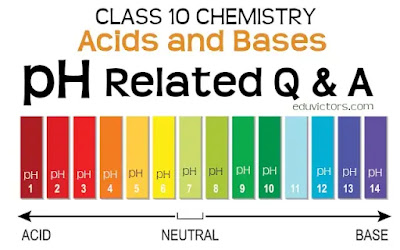# Carbon and Its Compounds - Very Short Answer Based Questions (VSQA)

Class 10 - Chemistry

Very Short Answer Based Questions (VSQA)

Q1: Define catenation.

Answer: The property of a carbon atom to link with other carbon atoms is known as catenation.

Q2: What is isomerism? .

Answer: Isomerism is a phenomenon in which compounds have the same molecular formula but different structural formula. ;

Q3: What are hydrocarbons?

Answer: The compounds containing carbon and hydrogen are known as hydrocarbons. For example, methane (CH₄).# Class 10 - Chemistry - Chemical Reactions and Equations (Q & A) Part-2

The first chapter of Chemistry talks about chemical reactions and equations. In a chemical reaction, reactants are transformed into products. In this series, the part-2 video covers questions and answers about the definition of chemical equation. Understand the difference between unbalanced and balanced chemical equations. Why balancing of chemical equation is necessary? Steps involved in balancing a chemical equation.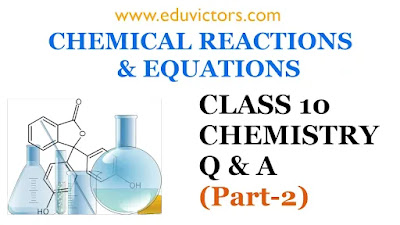## CBSE Class 10 - Chemistry - Chemical Reactions and Equations (Q & A) Part-1

The first chapter of Chemistry talks about chemical reactions and equations. In a chemical reaction, reactants are transformed into products. In this series, the part-1 video covers questions and answers about chemical changes or reactions. How do chemical changes differ from physical changes? Examples of chemical changes and indicators. What are the characteristics or indicators or chemical changes?

For Study Notes, Worksheets, Question Papers and Assignments for CBSE Class 6 - 12 check our website www.eduvictors.com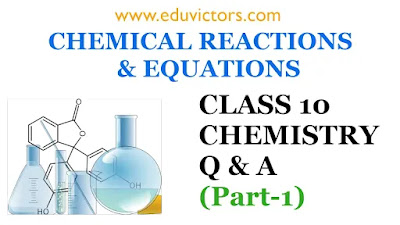## Understanding Chemical Changes

CHEMICAL REACTIONS AND EQUATIONS (Q & A)
CBSE Class 10 - Chemistry

Q1: What is a chemical change?

Answer: A chemical change is one in which a new substance is formed which has properties totally different from the starting materials. The starting materials are called as reactants and the new substances formed are called as products. In such changes, the nature and identity of the products is completely changed.

Q2: Give three examples of chemical change?## Science - MCQs

CBSE Class 10

Q1: Optical density of a medium depends on

(a) Density of the medium
(b) Thickness of the medium
(c) Velocity of light in that medium
(d) None of these

Q2: Which of the following is not a physical change?

(a) Boiling of water to give water vapour
(b) Melting of ice to give water
(c) Dissolution of salt in water
(d) Combustion of Liquefied Petroleum Gas (LPG)

Q3: Which of the following is correct about chromosomes?

(a) It is composed of DNA and lipids.
(b) It is composed of DNA and proteins.
(c) It is composed of DNA only.
(d) It is composed of RNA and proteins.## CBSE Class 10 - Chemistry - Assertion Reason Based Questions

Assertion and Reasoning based questions are a bit tricky. You should be able to distinguish whether the assertion is well connected to the reason or these are just random facts. Read both statements carefully, and check if both statements are factually or logically correct or not. If both are correct, then try to check if both are well connected to each other or not.

The questions below consist of statements of an Assertion and a Reason. Use the following key to choose an appropriate answer:

(A) If both assertion and reason are CORRECT and the reason is the CORRECT explanation of the assertion.
(B) If both assertion and reason are CORRECT, but the reason is NOT THE CORRECT explanation of the assertion.
(C) If assertion is CORRECT, but reason is INCORRECT
(D) If assertion is INCORRECT, but reason is CORRECT

QUESTIONS:

1.  ASSERTION:  Combustion reactions are also called exothermic oxidation reactions.
REASON:  In these reactions, oxygen is added and heat energy is released.## Class 10 Chemistry - Metals and Non-Metals

Multiple Choice Questions (MCQs)